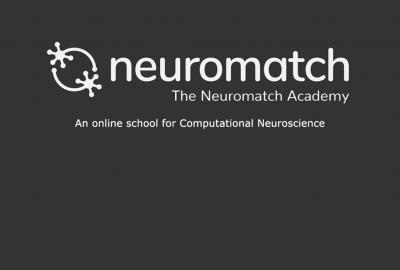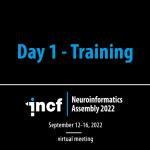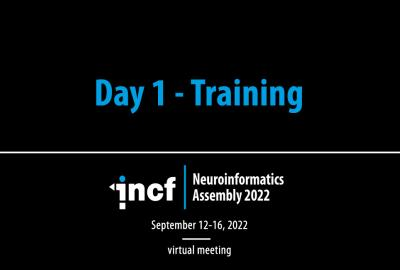# Linear Systems

Category
Level
Beginner

Neuromatch Academy aims to introduce traditional and emerging tools of computational neuroscience to trainees. It is appropriate for student population ranging from undergraduates to faculty in academic settings and also includes industry professionals. In addition to teaching the technical details of computational methods, Neuromatch Academy also provide a curriculum centered on modern neuroscience concepts taught by leading professors along with explicit instruction on how and why to apply models.

This course on introduces the dynamic features of the brain and their allied dynamical systems description including the twin perspectives from dynamical systems: geometric and algorithmic/computational. The course also covers linear dynamical systems in terms of approximations near equilibria and the usefulness of this approximation is in terms of geometrical approaches (eigenvector decompositions), including how this leads to line attractors and models of optimal decisions as well as “non-normal” surprises when eigenvectors are not orthogonal, as well as the treatment of time-dependent systems, including those driven by stochastic signals or noise, and close with examples on how networks and the activity that they produce co-evolve over time.

Course Features
Lectures
Videos
Tutorials
Slides
Recordings of question and answer sessions
Discussion forum on Neurostars.org
Lessons of this Course
1
1
Duration:
30:55

This lecture provides an introduction to linear systems.

2
2
Duration:
9:28

This tutorial covers the behavior of dynamical systems, systems that evolve in time, where the rules by which they evolve in time are described precisely by a differential equation.

Differential equations are equations that express the rate of change of the state variable 𝑥. One typically describes this rate of change using the derivative of 𝑥 with respect to time (𝑑𝑥/𝑑𝑡) on the left hand side of the differential equation: 𝑑𝑥𝑑𝑡=𝑓(𝑥). A common notational short-hand is to write 𝑥˙ for 𝑑𝑥𝑑𝑡. The dot means "the derivative with respect to time".

3
3
Duration:
3:24

This tutorial provides an introduction to the Markov process in a simple example where the state transitions are probabilistic. The aims of this tutorial is to help you understand Markov processes and history dependence, as well as to explore the behavior of a two-state telegraph process and understand how its equilibrium distribution is dependent on its parameters.

4
4
Duration:
2:54

This tutorial builds on how deterministic and stochastic processes can both be a part of a dynamical system by simulating random walks, investigating the mean and variance of a Ornstein-Uhlenbeck (OU) process, and quantifying the OU process's behavior at equilibrium.

5
5
Duration:
5:34

The goal of this tutorial is to use the modeling tools and intuitions developed in the previous few tutorials and use them to fit data. The concept is to flip the previous tutorial -- instead of generating synthetic data points from a known underlying process, what if we are given data points measured in time and have to learn the underlying process?

This tutorial is in two sections:

• Section 1 walks through using regression of data to solve for the coefficient of an OU process from Tutorial 3.
• Section 2 generalizes this auto-regression framework to high-order autoregressive models, and we will try to fit data from monkeys at typewriters.
6
6
Duration:
33:03

This lecture provides a summary of concepts associated with linear dynamical systems, covered in Linear Systems I (Intro Lecture) and Tutorials 1 - 4, and also introduces motor neuroscience/neuroengineering, brain-machine interfaces, and applications of dynamical systems.

##### Recent courses#### Reinforcement Learning#### INCF Assembly 2022 - Training Day 1